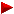HOME plateWON | World!OfNumbersDetails ofPalindromic Quasi_Over_SquaresPalindromic Quasi_Over_Squares

Legend
```
F_qs  Decomposition of the quasi_over_square in its prime factors.
Date  Date of discovery by the author.Hypertext links to related topics in number theory

```

Factorization
```F_qs  30.103 =
PRIME

Comm  30103 is the only known multi-digit palindromic prime
of the form sigma(n)/d(n), when n is composite. ( McCranie and Honaker )
sigma(n) = the sum of all the factors of n, including 1 and n itself.
d(n) = the number of factors of n, including 1 and n itself.

30103 is also nice because it comes up for
n = 149645 (an odd number) and once for
n = 179574 (an even number).

n         sigma(n)  d(n)  sigma(n)/d(n)=palprime
149645    180618    6     30103
179574    361236    12    30103

30103 is still the only "known" palprime with the property.

```

[TOP OF PAGE]

Patrick De Geest - Belgium- Short Bio - Some Pictures